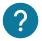# How a for loop works? How to use it in your program?

The for loop is used to repeat some instructions a determinate number of times. The for loop is really useful for repetitive action, such as blinking a led or move a servomotor from 0° to 180°.

``````for (initialization; condition; incrementation) {
}``````

As we can see, the for loop takes in parameters an initializing, a condition for which the loop is executed while the condition is true and an incrementation. A variable can be used in the loop which is initialized at a number and increment it every loop until the condition is false.

The incrementation is called the step. This is the value which your variable will be increment.### How to write this condition?

The for condition can be difficult to write with all the operators that exist!

We wrote a course about all the operators you can use in a for loop.

We will few examples of for loop below:

``````for (int i = 0; i < 255; i++) {
}``````

As we can see in our example, we use an i variable which is initialized at 0 and execute 255 times our instructions inside loop. The step is corresponds to i++, that ‘s mean that at each loop we will add 1 to variable i.

Nevertheless you can change the step that the loop finish quicker without executing 255 times. You can write for example i+=2 instead of i++. In this case the loop will execute 128 times.### Beware of how many times your loop will be executed!

If we use the previous example, the first value of i is 0 but it’s last value will be 254 et not 255 because we wrote lower (<) and not lower or equal (<=). I you change by lower or equal the condition of your loop you will have 256 steps in your loop because it will be from 0 to 255.

• For loop with decreasing incrementation

We will see now how to do a for loop in which the incrementation of the  variable is decreasing.

``````for (int i = 255; i > 0; i--) {